# Wandering interface method

The wandering interface method (WIM) is a general, versatile and robust procedure for the calculation of surface free energies . It can be employed to calculate liquid-vapor surface tension and solid-fluid interfacial tension alike. It does not require the explicit evaluation of the virial and may be employed for both continuous and discontinuous model potentials.

The method is based on a clear and appealing physical basis: since an interface has usually an extra energy cost, a system will try to stretch in order to minimize the interfacial area. The original feature of WIM is to allow the system's interfacial area to fluctuate freely and extract the surface free energy from the related surface area probability distribution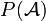$P({\mathcal A})$.

This goal is achieved in practice by employing a canonical ensemble where the box shape as described by the surface area,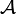${\mathcal A}$ is considered as an extra ensemble variable. During the course of the simulation, trial deformations of the simulation box at constant volume are attempted and either accepted or rejected according to the usual Metropolis Monte Carlo scheme. This provides a Markovian random walk of the interfacial area. Collecting the surface area probability distribution, one can readily calculate the surface free energy, since, according to statistical mechanics, one can show that: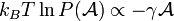$k_BT\ln P({\mathcal A}) \propto -\gamma {\mathcal A}$

Hence, the surface tension,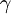$\gamma$ may be readily evaluated as the slope of$P({\mathcal A})$ on a logarithmic plot.

Since the system will tend to stretch continuously so as to achieve a vanishing surface area, one must constraint the extent of deformations in order to bracket the surface area fluctuations within a convenient interval. This is easily achieved in practice by considering a modified canonical ensemble where the microstate probability distribution is given by: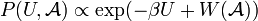$P(U,{\mathcal A}) \propto \exp(-\beta U + W({\mathcal A}) )$.

where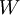$W$ is a biasing function chosen so as to constraint the fluctuations within some convenient interval. In the original implementation of WIM, the following biasing function was chosen: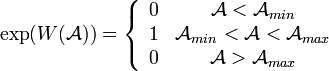$\exp(W({\mathcal A})) = \left \{ \begin{array}{cc} 0 & {\mathcal A} < {\mathcal A}_{min} \\ 1 & {\mathcal A}_{min} < {\mathcal A} < {\mathcal A}_{max} \\ 0 & {\mathcal A} > {\mathcal A}_{max} \end{array} \right .$

Several other choices are possible and have been tested since then.